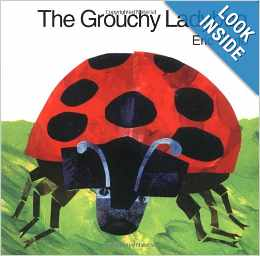# Practice Telling Time to the Hour and Half Hour

19 teachers like this lesson
Print Lesson

## Objective

SWBAT use the hour hand to draw and write times on analog and digital clocks.

#### Big Idea

It's about time! In this lesson students will practice telling time to the hour and half hour.

## Activating Strategy

10 minutes

To begin this lesson, I start by reading the book The Grouchy Ladybug by Eric Carle.  I start with this book because it discusses time throughout the book.  The pictures keep the students engaged and the story uses time as the story progresses.After reading the book, we then count by fives to 60.  You can see how we practice this in the video below.  I ask a student leader to use a pointed to point out the different numbers we are counting on either the hundreds grid or a number line.  Starting with a review of counting by 5s is to help to reinforce the concept of the clock being based on increments of fives.  We then review telling time to the hour and half hour using individual clocks.

The standard MD.B.3 requires that students write and tell time to the hour and half hour using analog and digital clocks.  When teaching this standard, it is important to furnish students with analog clocks for telling time.  They are exposed to various digital clocks on a daily basis, but less often to analog clocks.  In this lesson, they will practice writing the time using analog clocks.

## Teaching Strategies

15 minutes

In this lesson, students will practice telling time to the hour and half hour using a digital and analog clock.  Some children may ask why they need to learn how to tell time on an analog clock when most of the clocks they see are digital clocks. I explain that it is not enough to be able to look at a clock and know what time it is. I tell the students that understanding how the hands move around an analog clock is important because it helps to show how time passes. The movement of the hands helps to show why an hour is more time than a half hour, and a half hour is more time than a minute.

Understanding how the two hands of an analog clock work together to show the passing of time is a difficult concept for children to grasp. These introductory lessons help to build a foundation for later mastery of the concept of the passage of time.  After multiple experiences telling time to the hour and half hour, children may notice regularities (MP8) in positions of the hour and minute hands. Once they understand the process, children can generalize and create shortcuts in telling time.

To get them thinking about telling time, we practice telling time to the hour and half hour using individual clocks.  After reviewing the time, I display a clock and ask students to tell the time.  (located here: Practice Time to the Hour and Half Hour powerpoint.)  Have children look at the first set of clocks as you read the following problem aloud:

Barbara goes to the store at 8:00. Circle the clock that shows 8:00.

I then ask children the following questions to guide them in the solution process.

• Are you looking for a clock that shows a time to the hour or a time to the half hour? (time to the hour)
• What time does the first clock show? (8:30)
• How do you know the first clock shows 8:30? (The hour hand is halfway between the 8 and the 9, and the minute hand is pointing to the 6.)
• Does the second clock match the problem? Explain. (Yes; The second clock shows 8:00. The hour hand points to the 8, and the minute hand points to the 12.)Have children circle the second clock. Then have children look at the second set of clocks as you read the next problem aloud:

Barbara takes Ria for a walk at 1:30. Circle the clock that shows 1:30.

• Are you looking for a clock that shows a time to the hour or a time to the half hour? (time to the half hour)

Guide children to identify and circle the clock that shows 1:30.

I continue going through the next few slides as needed to reinforce the concept.

## Independent Practice

30 minutes

For the independent practice portion of this lesson, I hand out the worksheet (found here Practice Time to the Hour and Half Hour worksheet).  I like to circulate around the class as students work, to ensure their understanding.

For struggling students, you might want to pull them while the others are working and make sure they are solid on telling time to the hour. I do this by displaying an analog clock face that shows 6:00.

• What time does the clock show? (6:00
• How can you write the time on a digital clock? (Have a volunteer write the time on the board.)

Once I see that they are solid in this skill I extend the practice to include some times to the half hour, and if they show me that they are solid here too, I release them to complete the worksheet. Then I pull other small groups and show them analog clock faces on which a time to the half hour is shown for some extra practice with the teacher.

## Closing/Summarizing

5 minutes

To close out the lesson, I have students draw two clocks and show the time to the hour and half hour.  They use this as their "ticket out the door".